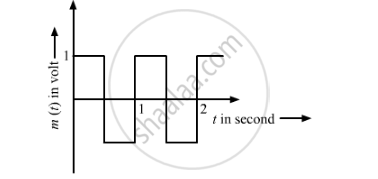# Find Modulation Index. - Physics

The carrier wave is given by

C(t) = 2sin(8πt) volt.

The modulating signal is a square wave as shown. Find modulation index.#### Solution

Modulation index (μ) is the ratio of the amplitude of the modulating signal to the amplitude of the carrier wave.

The generalised equation of a carrier wave is given below:
c(t) =Acsinωct

The generalised equation of a modulating wave is given below:

cm(t)=Acsinωct+μAcsinωmtsinωct

Here, μ is defined as A_m/A_c

On comparing this with the equations of carrier wave and modulating wave, we get:

Amplitude of modulating signal, Am=1 V

Amplitude of carrier wave, Ac=2 V

:.mu=A_m/A_c=1/2=0.5

Is there an error in this question or solution?
2013-2014 (March) Delhi Set 1

Share